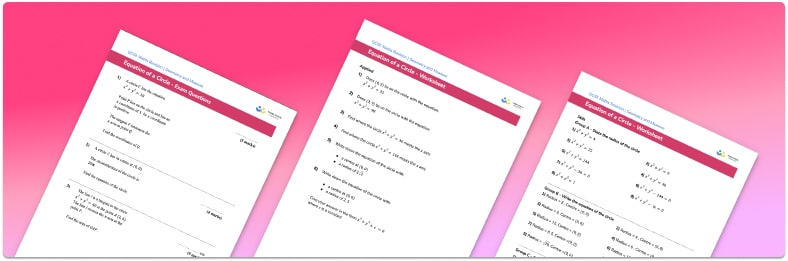# Equation Of A Circle Worksheet• Section 1 of the equation of a circle worksheet contains 27 skills-based equation of a circle questions, in 3 groups to support differentiation
• Section 2 contains 6 applied equation of a circle questions with a mix of worded problems and deeper problem solving questions
• Section 3 contains 3 foundation and higher level GCSE exam style questions
• Section 1 of the equation of a circle worksheet contains 27 skills-based equation of a circle questions, in 3 groups to support differentiation
• Section 2 contains 6 applied equation of a circle questions with a mix of worded problems and deeper problem solving questions
• Section 3 contains 3 foundation and higher level GCSE exam style questions
• Answers and a mark scheme for all questions are provided
• Questions follow variation theory with plenty of opportunities for students to work independently at their own level
• All questions created by fully qualified expert secondary maths teachers
• Suitable for GCSE maths revision for AQA, OCR and Edexcel exam boards

style=”font-weight: 400;”>Answers and a mark scheme for all questions are provided

• Questions follow variation theory with plenty of opportunities for students to work independently at their own level
• All questions created by fully qualified expert secondary maths teachers
• Suitable for GCSE maths revision for AQA, OCR and Edexcel exam boards

• This field is for validation purposes and should be left unchanged.

You can unsubscribe at any time (each email we send will contain an easy way to unsubscribe). To find out more about how we use your data, see our privacy policy.

### Equation of a circle at a glance

The general equation of a circle is part of the topic on non-linear equations and graphs in coordinate geometry. Students are expected to recognise the equation of a circle in the form: x²+y²=r², where r is the radius of the circle.

The general equation of the circle in centre-radius form, (x+a)²+(y+b)²=, is not examined at GCSE; the centre of the circle will always be the origin when the circle graph is plotted on the xy coordinate plane.

Students may be asked to find the coordinates of the point(s) where the circle crosses an axis. For example, to find the point(s) where the circle x²+y²=9 crosses the y-axis, substitute x=0 into the equation of the circle, then solve the resulting quadratic equation.

A tangent to a circle is a straight line that touches the circle at one point. The tangent is perpendicular to the radius of the circle. To find the equation of the tangent at this point, first find the gradient of the radius, then use this to find the gradient of the tangent. Finally, find the equation of the tangent using its gradient and the known point on the circumference, and write in the standard form y=mx+c.

Looking forward, students can then progress to additional circles, sectors and arcs worksheets and other geometry worksheets, for example an angles in polygons worksheet or area and circumference of a circle worksheet.For more teaching and learning support on Geometry our GCSE maths lessons provide step by step support for all GCSE maths concepts.

## Do you have KS4 students who need more focused attention to succeed at GCSE?There will be students in your class who require individual attention to help them succeed in their maths GCSEs. In a class of 30, it’s not always easy to provide.

Help your students feel confident with exam-style questions and the strategies they’ll need to answer them correctly with our dedicated GCSE maths revision programme.

Lessons are selected to provide support where each student needs it most, and specially-trained GCSE maths tutors adapt the pitch and pace of each lesson. This ensures a personalised revision programme that raises grades and boosts confidence.

Find out more